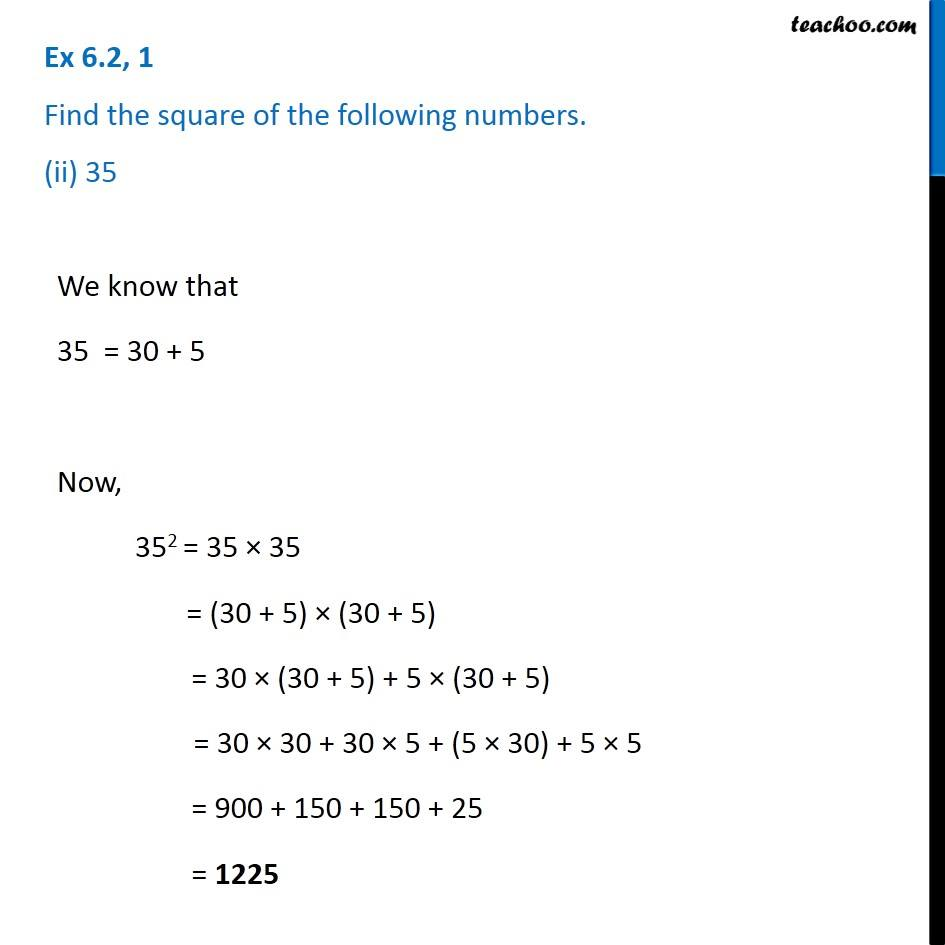Ex 6.2

Chapter 6 Class 8 Squares and Square Roots
Serial order wise### Transcript

Ex 6.2, 1 Find the square of the following numbers. (ii) 35We know that 35 = 30 + 5 Now, 352 = 35 × 35 = (30 + 5) × (30 + 5) = 30 × (30 + 5) + 5 × (30 + 5) = 30 × 30 + 30 × 5 + (5 × 30) + 5 × 5 = 900 + 150 + 150 + 25 = 1225